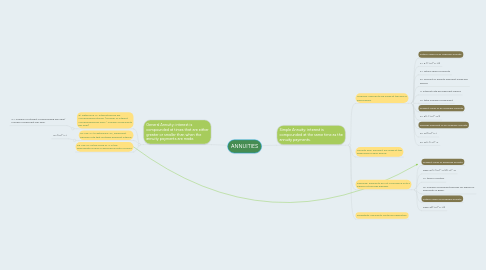# ANNUITIES

Jetzt loslegen. Gratis!ANNUITIES## 1. Simple Annuity: interest is compounded at the same time as the annuity payments.

### 1.1. Ordinary: Payments are made at the end of each period.

1.1.1. Future Value of an Ordinary Annuity

1.1.2. F= R ( (1+i)^n-1/i)

1.1.3. F= future value of annuity

1.1.4. R= amount of annuity payment made per period

1.1.5. i= interest rate per payment period

1.1.6. n= total number of payment

1.1.7. Present Value of an Ordinary Annuity

1.1.8. P= R(1-(1+i)^-n/i)

1.1.9. Periodic Payment of an Ordinary Annuity

1.1.10. R= Fi/(1+i)^n-1

1.1.11. R= Pi/1-(1+i)^-n

### 1.3. Deferred: Payments do not commence until a period of time has elapsed.

1.3.1. Present Value of Deferred Annuity

1.3.2. Pdef=R(1-(1+i)^-n/i)(1+i)^-d

1.3.3. n= time in months

1.3.4. d= number of payment periods for which no payments is given.

1.3.5. Future Value of Deferred Annuity

1.3.6. Fdef=R((1+i)^n-1/i)

## 2. General Annuity: interest is compounded at times that are either greater or smaller than when the annuity payments are made.

### 2.1. #1 Determine "C" interest period per compounding interval. (number of interest compounding per year /  number of payments per year)

2.1.1. C= number of interest compounding per year/ number of payment per year.

### 2.2. #2 Use "C" to determine "i2", equivalent periodic rate that matches payment interval.

2.2.1. i2=(1+i)^c-1